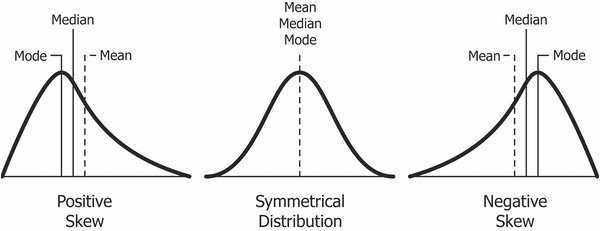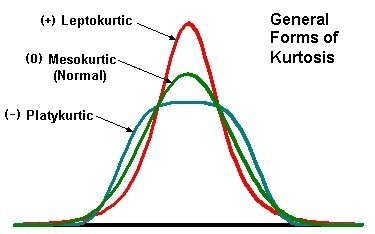# Machine Learning: Notes and Advice

##### Norms
• L0 - The total number of nonzero elements in a vector
• L1 - Manhattan Distance
• L2 - Euclidean distance
• L - Maximum of absolute values of all the elements of the vector
##### Am I overfitting/underfitting?
• Training loss much greater than validation loss. That is underfitting.
• Training loss much less than validation loss. That is overfitting.
##### Ensemble methods
• Bagging - Sample the data a bunch of times, and train a model on each same, then aggregate the model's outputs (ex. random forest)
• Boosting - Feed the output of one model into the input of another model (ex. haar cascade)
• Stacking - Train different types of models on the data, then aggregate their outputs (ex. I wanna win the kaggle competition/Netflix prize)
##### Bias vs Variance
• Bias - Your bias about people is your assumptions about people. If you have high bias, you have incorrect assumptions. Generally: High bias = underfitting
• Variance - How much do you vary depending on the dataset? How much do you fit the noise? Generally: High variance = overfitting
##### Good ideas to improve a model
• Cluster the unlabeled training data, then add cluster features to get additional free features
• Let's say you have multiple labels per data point in your training data. If labelers disagree a lot on a single data point, then that sample is pretty crappy. If you sort your data in order of crappiness, then drop the most crappy ones first, then you'll get a small boost in model performance. Even smart is to penalize the model less if it misclassifies a crappier data point.
##### Multi-class vs multi-label classification
• Multi-class classification: 1 category per data point
• Multi-label classification: >1 category per data point
##### Precision vs Recall (w/ statistics translation)##### Statistics##### Type I vs Type II errors